# 神经网络变得轻松（第八部分）：关注机制

3 三月 2021, 09:40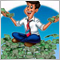0
686

### 1. 关注机制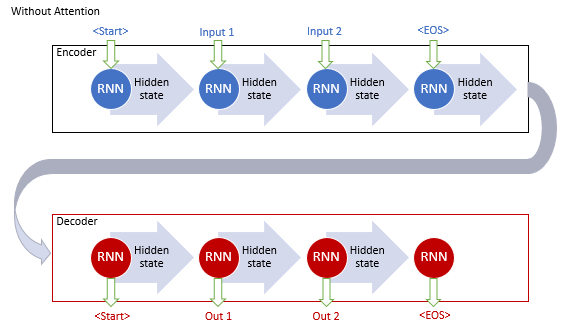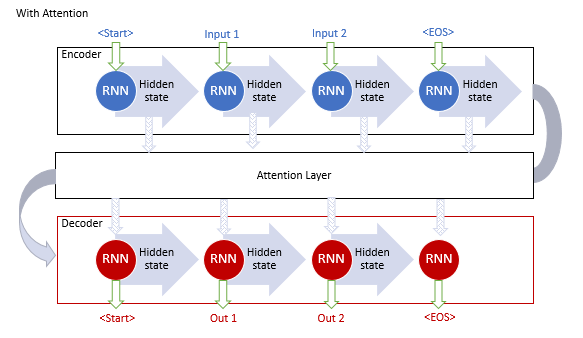1. 创建编码器的隐藏状态，并将其累积在关注模块当中。

2. 评估每个编码器元素的隐藏状态与解码器最后一个隐藏状态之间的配对依赖性。

3. 将结果得分合并为一个向量，并利用 Softmax 函数对其进行常规化。

4. 所有编码器的隐藏状态乘以它们对应的对齐分数，以便计算上下文向量。

5. 解码上下文向量，并将结果值与解码器的先前状态组合。

### 2. 自关注机制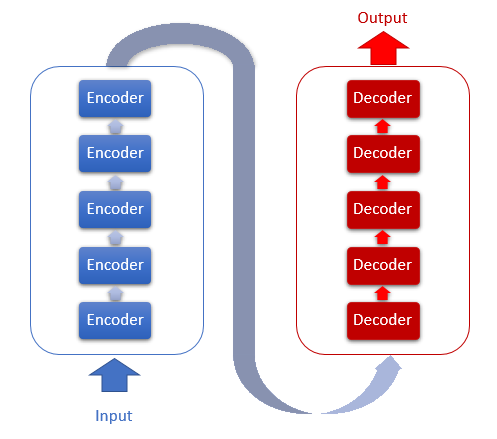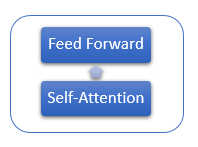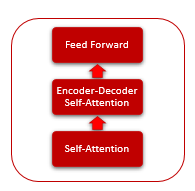1. 首先，我们计算 Query、Key 和 Value 向量。 这些向量由序列的每个元素乘以相应的矩阵 WQ、WK 和 WV 得到的。

2. 接着，判断序列元素之间的配对依赖性。 为此，将 Query 向量乘以序列中所有元素的 Key 向量。 重复迭代序列中每个元素的 Query 向量。 迭代的结果就是，我们得到大小为 N*N 的 Score 矩阵，其中 N 是序列的大小。

3. 下一步是在每个 Query 的上下文中，将结果值除以 Key 向量维度的平方根，并利用 Softmax 函数对其进行常规化。 由此，我们得到了序列元素之间的配对依赖系数。

4. 将每个 Value 向量乘以相应的依赖系数，从而得到调整后的元素值。 此迭代的目的是聚焦于相关元素，并降低不相关数值的影响。

5. 接下来，把每个元素的所有调整后的 Value 向量汇总。 该操作的结果将是“自关注”层的输出值向量。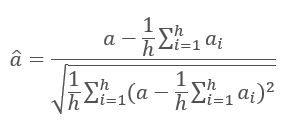[ 11 ] 文章中更详细地讨论了神经网络层的常规化。

### 3. 实现

#### 3.1. 升级卷积层

```class CNeuronConvOCL    :  public CNeuronProofOCL
{
protected:
uint              iWindowOut;
//---
CBufferDouble     *WeightsConv;
CBufferDouble     *DeltaWeightsConv;
CBufferDouble     *FirstMomentumConv;
CBufferDouble     *SecondMomentumConv;
//---
virtual bool      feedForward(CNeuronBaseOCL *NeuronOCL);
virtual bool      updateInputWeights(CNeuronBaseOCL *NeuronOCL);

public:
CNeuronConvOCL(void) :   iWindowOut(1) {  activation=LReLU;   }
~CNeuronConvOCL(void);
virtual bool      Init(uint numOutputs,uint myIndex,COpenCLMy *open_cl,uint window, uint step, uint window_out, uint units_count, ENUM_OPTIMIZATION optimization_type);
//---
virtual bool      SetGradientIndex(int index)   {  return Gradient.BufferSet(index);   }
//---
virtual bool      calcInputGradients(CNeuronBaseOCL *NeuronOCL);
virtual int       Type(void)   const   {  return defNeuronConvOCL;   }
//--- methods for working with files
virtual bool      Save(int const file_handle);
virtual bool      Load(int const file_handle);
};
```

```__kernel void FeedForwardConv(__global double *matrix_w,
__global double *matrix_i,
__global double *matrix_o,
int inputs, int step,
int window_in, int window_out,
uint activation)
{
int i=get_global_id(0);
int w_in=window_in;
int w_out=window_out;
double sum=0.0;
double4 inp, weight;
int shift_out=w_out*i;
int shift_in=step*i;
for(int out=0;out<w_out;out++)
{
int shift=(w_in+1)*out;
int stop=(w_in<=(inputs-shift_in) ? w_in : (inputs-shift_in));
for(int k=0; k<=stop; k=k+4)
{
switch(stop-k)
{
case 0:
inp=(double4)(1,0,0,0);
weight=(double4)(matrix_w[shift+k],0,0,0);
break;
case 1:
inp=(double4)(matrix_i[shift_in+k],1,0,0);
weight=(double4)(matrix_w[shift+k],matrix_w[shift+k+1],0,0);
break;
case 2:
inp=(double4)(matrix_i[shift_in+k],matrix_i[shift_in+k+1],1,0);
weight=(double4)(matrix_w[shift+k],matrix_w[shift+k+1],matrix_w[shift+k+2],0);
break;
case 3:
inp=(double4)(matrix_i[shift_in+k],matrix_i[shift_in+k+1],matrix_i[shift_in+k+2],1);
weight=(double4)(matrix_w[shift+k],matrix_w[shift+k+1],matrix_w[shift+k+2],matrix_w[shift+k+3]);
break;
default:
inp=(double4)(matrix_i[shift_in+k],matrix_i[shift_in+k+1],matrix_i[shift_in+k+2],matrix_i[shift_in+k+3]);
weight=(double4)(matrix_w[shift+k],matrix_w[shift+k+1],matrix_w[shift+k+2],matrix_w[shift+k+3]);
break;
}
sum+=dot(inp,weight);
}
switch(activation)
{
case 0:
sum=tanh(sum);
break;
case 1:
sum=1/(1+exp(-clamp(sum,-50.0,50.0)));
break;
case 2:
if(sum<0)
sum*=0.01;
break;
default:
break;
}
matrix_o[out+shift_out]=sum;
}
}
```

```bool CNeuronConvOCL::feedForward(CNeuronBaseOCL *NeuronOCL)
{
if(CheckPointer(OpenCL)==POINTER_INVALID || CheckPointer(NeuronOCL)==POINTER_INVALID)
return false;
uint global_work_offset={0};
uint global_work_size;
global_work_size=Output.Total()/iWindowOut;
OpenCL.SetArgumentBuffer(def_k_FeedForwardConv,def_k_ffc_matrix_w,WeightsConv.GetIndex());
OpenCL.SetArgumentBuffer(def_k_FeedForwardConv,def_k_ffc_matrix_i,NeuronOCL.getOutputIndex());
OpenCL.SetArgumentBuffer(def_k_FeedForwardConv,def_k_ffc_matrix_o,Output.GetIndex());
OpenCL.SetArgument(def_k_FeedForwardConv,def_k_ffc_inputs,NeuronOCL.Neurons());
OpenCL.SetArgument(def_k_FeedForwardConv,def_k_ffc_step,iStep);
OpenCL.SetArgument(def_k_FeedForwardConv,def_k_ffc_window_in,iWindow);
OpenCL.SetArgument(def_k_FeedForwardConv,def_k_ffс_window_out,iWindowOut);
OpenCL.SetArgument(def_k_FeedForwardConv,def_k_ffc_activation,(int)activation);
if(!OpenCL.Execute(def_k_FeedForwardConv,1,global_work_offset,global_work_size))
{
printf("Error of execution kernel FeedForwardProof: %d",GetLastError());
return false;
}
//---
return Output.BufferRead();
}
```

#### 3.2. 自关注模块类

```class CNeuronAttentionOCL : public CNeuronBaseOCL
{
protected:
CNeuronConvOCL    *Querys;
CNeuronConvOCL    *Keys;
CNeuronConvOCL    *Values;
CBufferDouble     *Scores;
CNeuronBaseOCL    *AttentionOut;
CNeuronConvOCL    *FF1;
CNeuronConvOCL    *FF2;
//---
uint              iWindow;
uint              iUnits;
//---
virtual bool      feedForward(CNeuronBaseOCL *prevLayer);
virtual bool      updateInputWeights(CNeuronBaseOCL *prevLayer);

public:
CNeuronAttentionOCL(void) : iWindow(1), iUnits(0) {};
~CNeuronAttentionOCL(void);
virtual bool      Init(uint numOutputs,uint myIndex,COpenCLMy *open_cl, uint window, uint units_count, ENUM_OPTIMIZATION optimization_type);
virtual bool      calcInputGradients(CNeuronBaseOCL *prevLayer);
//---
virtual int       Type(void)   const   {  return defNeuronAttentionOCL;   }
//--- methods for working with files
virtual bool      Save(int const file_handle);
virtual bool      Load(int const file_handle);
};

```

```bool CNeuronAttentionOCL::Init(uint numOutputs,uint myIndex,COpenCLMy *open_cl,uint window,uint units_count,ENUM_OPTIMIZATION optimization_type)
{
if(!CNeuronBaseOCL::Init(numOutputs,myIndex,open_cl,units_count*window,optimization_type))
return false;
```

```//---
if(CheckPointer(Querys)==POINTER_INVALID)
{
Querys=new CNeuronConvOCL();
if(CheckPointer(Querys)==POINTER_INVALID)
return false;
if(!Querys.Init(0,0,open_cl,window,window,window,units_count,optimization_type))
return false;
Querys.SetActivationFunction(TANH);
}
//---
if(CheckPointer(Keys)==POINTER_INVALID)
{
Keys=new CNeuronConvOCL();
if(CheckPointer(Keys)==POINTER_INVALID)
return false;
if(!Keys.Init(0,1,open_cl,window,window,window,units_count,optimization_type))
return false;
Keys.SetActivationFunction(TANH);
}
//---
if(CheckPointer(Values)==POINTER_INVALID)
{
Values=new CNeuronConvOCL();
if(CheckPointer(Values)==POINTER_INVALID)
return false;
if(!Values.Init(0,2,open_cl,window,window,window,units_count,optimization_type))
return false;
Values.SetActivationFunction(None);
}
```

```   if(CheckPointer(Scores)==POINTER_INVALID)
{
Scores=new CBufferDouble();
if(CheckPointer(Scores)==POINTER_INVALID)
return false;
}
if(!Scores.BufferInit(units_count*units_count,0.0))
return false;
if(!Scores.BufferCreate(OpenCL))
return false;
```

```   if(CheckPointer(AttentionOut)==POINTER_INVALID)
{
AttentionOut=new CNeuronBaseOCL();
if(CheckPointer(AttentionOut)==POINTER_INVALID)
return false;
if(!AttentionOut.Init(0,3,open_cl,window*units_count,optimization_type))
return false;
AttentionOut.SetActivationFunction(None);
}

```

```   if(CheckPointer(FF1)==POINTER_INVALID)
{
FF1=new CNeuronConvOCL();
if(CheckPointer(FF1)==POINTER_INVALID)
return false;
if(!FF1.Init(0,4,open_cl,window,window,window*2,units_count,optimization_type))
return false;
FF1.SetActivationFunction(LReLU);
}
//---
if(CheckPointer(FF2)==POINTER_INVALID)
{
FF2=new CNeuronConvOCL();
if(CheckPointer(FF2)==POINTER_INVALID)
return false;
if(!FF2.Init(0,5,open_cl,window*2,window*2,window,units_count,optimization_type))
return false;
FF2.SetActivationFunction(None);
FF2.SetGradientIndex(Gradient.GetIndex());
}

```

```   iWindow=window;
iUnits=units_count;
activation=FF2.Activation();
//---
return true;
}
```

#### 3.3. 自关注前馈

```bool CNeuronAttentionOCL::feedForward(CNeuronBaseOCL *prevLayer)
{
if(CheckPointer(prevLayer)==POINTER_INVALID)
return false;

```

```  {
uint global_work_offset={0};
uint global_work_size;
global_work_size=1;
OpenCL.SetArgumentBuffer(def_k_Normilize,def_k_norm_buffer,prevLayer.getOutputIndex());
OpenCL.SetArgument(def_k_Normilize,def_k_norm_dimension,prevLayer.Neurons());
if(!OpenCL.Execute(def_k_Normilize,1,global_work_offset,global_work_size))
{
printf("Error of execution kernel Normalize: %d",GetLastError());
return false;
}
if(!prevLayer.Output.BufferRead())
return false;
}

```

```__kernel void Normalize(__global double *buffer,
int dimension)
{
int n=get_global_id(0);
int shift=n*dimension;
double mean=0;
for(int i=0;i<dimension;i++)
mean+=buffer[shift+i];
mean/=dimension;
double variance=0;
for(int i=0;i<dimension;i++)
variance+=pow(buffer[shift+i]-mean,2);
variance=sqrt(variance/dimension);
for(int i=0;i<dimension;i++)
buffer[shift+i]=(buffer[shift+i]-mean)/(variance==0 ? 1 : variance);
}
```

```   if(CheckPointer(Querys)==POINTER_INVALID || !Querys.FeedForward(prevLayer))
return false;
if(CheckPointer(Keys)==POINTER_INVALID || !Keys.FeedForward(prevLayer))
return false;
if(CheckPointer(Values)==POINTER_INVALID || !Values.FeedForward(prevLayer))
return false;

```

```  {
uint global_work_offset={0};
uint global_work_size;
global_work_size=iUnits;
OpenCL.SetArgumentBuffer(def_k_AttentionScore,def_k_as_querys,Querys.getOutputIndex());
OpenCL.SetArgumentBuffer(def_k_AttentionScore,def_k_as_keys,Keys.getOutputIndex());
OpenCL.SetArgumentBuffer(def_k_AttentionScore,def_k_as_score,Scores.GetIndex());
OpenCL.SetArgument(def_k_AttentionScore,def_k_as_dimension,iWindow);
if(!OpenCL.Execute(def_k_AttentionScore,1,global_work_offset,global_work_size))
{
printf("Error of execution kernel AttentionScore: %d",GetLastError());
return false;
}
if(!Scores.BufferRead())
return false;
}

```

```__kernel void AttentionScore(__global double *querys,
__global double *keys,
__global double *score,
int dimension)
{
int q=get_global_id(0);
int shift_q=q*dimension;
int units=get_global_size(0);
int shift_s=q*units;
double koef=sqrt((double)(units*dimension));
if(koef<1)
koef=1;
double sum=0;
for(int k=0;k<units;k++)
{
double result=0;
int shift_k=k*dimension;
for(int i=0;i<dimension;i++)
result+=(querys[shift_q+i]*keys[shift_k+i]);
result=exp(result/koef);
score[shift_s+k]=result;
sum+=result;
}
for(int k=0;k<units;k++)
score[shift_s+k]/=sum;
}
```

```  {
uint global_work_offset={0,0};
uint global_work_size;
global_work_size=iUnits;
global_work_size=iWindow;
OpenCL.SetArgumentBuffer(def_k_AttentionOut,def_k_aout_scores,Scores.GetIndex());
OpenCL.SetArgumentBuffer(def_k_AttentionOut,def_k_aout_inputs,prevLayer.getOutputIndex());
OpenCL.SetArgumentBuffer(def_k_AttentionOut,def_k_aout_values,Values.getOutputIndex());
OpenCL.SetArgumentBuffer(def_k_AttentionOut,def_k_aout_out,AttentionOut.getOutputIndex());
if(!OpenCL.Execute(def_k_AttentionOut,2,global_work_offset,global_work_size))
{
printf("Error of execution kernel Attention Out: %d",GetLastError());
return false;
}
double temp[];
if(!AttentionOut.getOutputVal(temp))
return false;
}

```

```__kernel void AttentionOut(__global double *scores,
__global double *values,
__global double *inputs,
__global double *out)
{
int units=get_global_size(0);
int u=get_global_id(0);
int d=get_global_id(1);
int dimension=get_global_size(1);
int shift=u*dimension+d;
double result=0;
for(int i=0;i<units;i++)
result+=scores[u*units+i]*values[i*dimension+d];
out[shift]=result+inputs[shift];
}
```

```  {
uint global_work_offset={0};
uint global_work_size;
global_work_size=1;
OpenCL.SetArgumentBuffer(def_k_Normilize,def_k_norm_buffer,AttentionOut.getOutputIndex());
OpenCL.SetArgument(def_k_Normilize,def_k_norm_dimension,AttentionOut.Neurons());
if(!OpenCL.Execute(def_k_Normilize,1,global_work_offset,global_work_size))
{
printf("Error of execution kernel Normalize: %d",GetLastError());
return false;
}
double temp[];
if(!AttentionOut.getOutputVal(temp))
return false;
}

```

```   if(!FF1.FeedForward(AttentionOut))
return false;
if(!FF2.FeedForward(FF1))
return false;

```

```  {
uint global_work_offset={0};
uint global_work_size;
global_work_size=iUnits;
OpenCL.SetArgumentBuffer(def_k_MatrixSum,def_k_sum_matrix1,AttentionOut.getOutputIndex());
OpenCL.SetArgumentBuffer(def_k_MatrixSum,def_k_sum_matrix2,FF2.getOutputIndex());
OpenCL.SetArgumentBuffer(def_k_MatrixSum,def_k_sum_matrix_out,Output.GetIndex());
OpenCL.SetArgument(def_k_MatrixSum,def_k_sum_dimension,iWindow);
if(!OpenCL.Execute(def_k_MatrixSum,1,global_work_offset,global_work_size))
{
printf("Error of execution kernel MatrixSum: %d",GetLastError());
return false;
}
if(!Output.BufferRead())
return false;
}
//---
return true;
}

```

```__kernel void SumMatrix(__global double *matrix1,
__global double *matrix2,
__global double *matrix_out,
int dimension)
{
const int i=get_global_id(0)*dimension;
for(int k=0;k<dimension;k++)
matrix_out[i+k]=matrix1[i+k]+matrix2[i+k];
}

```

#### 3.4. 自关注反馈

```bool CNeuronAttentionOCL::calcInputGradients(CNeuronBaseOCL *prevLayer)
{
if(CheckPointer(prevLayer)==POINTER_INVALID)
return false;
//---
if(!FF2.calcInputGradients(FF1))
return false;
if(!FF1.calcInputGradients(AttentionOut))
return false;

```

```  {
uint global_work_offset={0};
uint global_work_size;
global_work_size=iUnits;
OpenCL.SetArgumentBuffer(def_k_MatrixSum,def_k_sum_matrix1,AttentionOut.getGradientIndex());
OpenCL.SetArgumentBuffer(def_k_MatrixSum,def_k_sum_matrix2,Gradient.GetIndex());
OpenCL.SetArgumentBuffer(def_k_MatrixSum,def_k_sum_matrix_out,AttentionOut.getGradientIndex());
OpenCL.SetArgument(def_k_MatrixSum,def_k_sum_dimension,iWindow);
if(!OpenCL.Execute(def_k_MatrixSum,1,global_work_offset,global_work_size))
{
printf("Error of execution kernel MatrixSum: %d",GetLastError());
return false;
}
double temp[];
if(AttentionOut.getGradient(temp)<=0)
return false;
}

```

```  {
uint global_work_offset={0,0};
uint global_work_size;
global_work_size=iUnits;
global_work_size=iWindow;
OpenCL.SetArgumentBuffer(def_k_AttentionGradients,def_k_ag_gradient,AttentionOut.getGradientIndex());
OpenCL.SetArgumentBuffer(def_k_AttentionGradients,def_k_ag_keys,Keys.getOutputIndex());
OpenCL.SetArgumentBuffer(def_k_AttentionGradients,def_k_ag_keys_g,Keys.getGradientIndex());
OpenCL.SetArgumentBuffer(def_k_AttentionGradients,def_k_ag_querys,Querys.getOutputIndex());
OpenCL.SetArgumentBuffer(def_k_AttentionGradients,def_k_ag_querys_g,Querys.getGradientIndex());
OpenCL.SetArgumentBuffer(def_k_AttentionGradients,def_k_ag_values,Values.getOutputIndex());
OpenCL.SetArgumentBuffer(def_k_AttentionGradients,def_k_ag_values_g,Values.getGradientIndex());
OpenCL.SetArgumentBuffer(def_k_AttentionGradients,def_k_ag_scores,Scores.GetIndex());
if(!OpenCL.Execute(def_k_AttentionGradients,2,global_work_offset,global_work_size))
{
printf("Error of execution kernel AttentionGradients: %d",GetLastError());
return false;
}
double temp[];
if(Keys.getGradient(temp)<=0)
return false;
}

```

```__kernel void AttentionIsideGradients(__global double *querys,__global double *querys_g,
__global double *keys,__global double *keys_g,
__global double *values,__global double *values_g,
__global double *scores,
__global double *gradient)
{
int u=get_global_id(0);
int d=get_global_id(1);
int units=get_global_size(0);
int dimension=get_global_size(1);
double koef=sqrt((double)(units*dimension));
if(koef<1)
koef=1;
//---
double vg=0;
double qg=0;
double kg=0;
for(int iu=0;iu<units;iu++)
{
double g=gradient[iu*dimension+d]/2;
double sc=scores[iu*units+u];
vg+=sc*g;

```

```//---
double sqg=0;
double skg=0;
for(int id=0;id<dimension;id++)
{
sqg+=values[iu*dimension+id]*gradient[u*dimension+id]/2;
skg+=values[u*dimension+id]*gradient[iu*dimension+id]/2;
}
qg+=(scores[u*units+iu]==0 || scores[u*units+iu]==1 ? 0.0001 : scores[u*units+iu]*(1-scores[u*units+iu]))*sqg*keys[iu*dimension+d]/koef;
//---
kg+=(scores[iu*units+u]==0 || scores[iu*units+u]==1 ? 0.0001 : scores[iu*units+u]*(1-scores[iu*units+u]))*skg*querys[iu*dimension+d]/koef;
}
int shift=u*dimension+d;
values_g[shift]=vg;
querys_g[shift]=qg;
keys_g[shift]=kg;
}

```

```   if(!Querys.calcInputGradients(prevLayer))
return false;
//---
{
uint global_work_offset={0};
uint global_work_size;
global_work_size=iUnits;
OpenCL.SetArgumentBuffer(def_k_MatrixSum,def_k_sum_matrix1,AttentionOut.getGradientIndex());
OpenCL.SetArgumentBuffer(def_k_MatrixSum,def_k_sum_matrix2,prevLayer.getGradientIndex());
OpenCL.SetArgumentBuffer(def_k_MatrixSum,def_k_sum_matrix_out,AttentionOut.getGradientIndex());
OpenCL.SetArgument(def_k_MatrixSum,def_k_sum_dimension,iWindow);
if(!OpenCL.Execute(def_k_MatrixSum,1,global_work_offset,global_work_size))
{
printf("Error of execution kernel MatrixSum: %d",GetLastError());
return false;
}
double temp[];
if(AttentionOut.getGradient(temp)<=0)
return false;
}
//---
if(!Keys.calcInputGradients(prevLayer))
return false;
//---
{
uint global_work_offset={0};
uint global_work_size;
global_work_size=iUnits;
OpenCL.SetArgumentBuffer(def_k_MatrixSum,def_k_sum_matrix1,AttentionOut.getGradientIndex());
OpenCL.SetArgumentBuffer(def_k_MatrixSum,def_k_sum_matrix2,prevLayer.getGradientIndex());
OpenCL.SetArgumentBuffer(def_k_MatrixSum,def_k_sum_matrix_out,AttentionOut.getGradientIndex());
OpenCL.SetArgument(def_k_MatrixSum,def_k_sum_dimension,iWindow);
if(!OpenCL.Execute(def_k_MatrixSum,1,global_work_offset,global_work_size))
{
printf("Error of execution kernel MatrixSum: %d",GetLastError());
return false;
}
double temp[];
if(AttentionOut.getGradient(temp)<=0)
return false;
}
//---
if(!Values.calcInputGradients(prevLayer))
return false;
//---
{
uint global_work_offset={0};
uint global_work_size;
global_work_size=iUnits;
OpenCL.SetArgumentBuffer(def_k_MatrixSum,def_k_sum_matrix1,AttentionOut.getGradientIndex());
OpenCL.SetArgumentBuffer(def_k_MatrixSum,def_k_sum_matrix2,prevLayer.getGradientIndex());
OpenCL.SetArgumentBuffer(def_k_MatrixSum,def_k_sum_matrix_out,prevLayer.getGradientIndex());
OpenCL.SetArgument(def_k_MatrixSum,def_k_sum_dimension,iWindow+1);
if(!OpenCL.Execute(def_k_MatrixSum,1,global_work_offset,global_work_size))
{
printf("Error of execution kernel MatrixSum: %d",GetLastError());
return false;
}
double temp[];
if(prevLayer.getGradient(temp)<=0)
return false;
}
//---
{
uint global_work_offset={0};
uint global_work_size;
global_work_size=1;
OpenCL.SetArgumentBuffer(def_k_Normilize,def_k_norm_buffer,prevLayer.getGradientIndex());
OpenCL.SetArgument(def_k_Normilize,def_k_norm_dimension,prevLayer.Neurons());
if(!OpenCL.Execute(def_k_Normilize,1,global_work_offset,global_work_size))
{
printf("Error of execution kernel Normalize: %d",GetLastError());
return false;
}
double temp[];
if(prevLayer.getGradient(temp)<=0)
return false;
}
//---
return true;
}
```

```bool CNeuronAttentionOCL::updateInputWeights(CNeuronBaseOCL *prevLayer)
{
if(!Querys.UpdateInputWeights(prevLayer))
return false;
if(!Keys.UpdateInputWeights(prevLayer))
return false;
if(!Values.UpdateInputWeights(prevLayer))
return false;
if(!FF1.UpdateInputWeights(AttentionOut))
return false;
if(!FF2.UpdateInputWeights(FF1))
return false;
//---
return true;
}

```

#### 3.5. 神经网络基类的变化

```#define def_k_FeedForwardConv    7
#define def_k_ffc_matrix_w       0
#define def_k_ffc_matrix_i       1
#define def_k_ffc_matrix_o       2
#define def_k_ffc_inputs         3
#define def_k_ffc_step           4
#define def_k_ffc_window_in      5
#define def_k_ffс_window_out     6
#define def_k_ffc_activation     7
//---
#define def_k_CalcHiddenGradientConv 8
#define def_k_chgc_matrix_w      0
#define def_k_chgc_matrix_g      1
#define def_k_chgc_matrix_o      2
#define def_k_chgc_matrix_ig     3
#define def_k_chgc_outputs       4
#define def_k_chgc_step          5
#define def_k_chgc_window_in     6
#define def_k_chgc_window_out    7
#define def_k_chgc_activation    8
//---
#define def_k_UpdateWeightsConvMomentum      9
#define def_k_uwcm_matrix_w       0
#define def_k_uwcm_matrix_g       1
#define def_k_uwcm_matrix_i       2
#define def_k_uwcm_matrix_dw      3
#define def_k_uwcm_inputs         4
#define def_k_uwcm_learning_rates 5
#define def_k_uwcm_momentum       6
#define def_k_uwcm_window_in      7
#define def_k_uwcm_window_out     8
#define def_k_uwcm_step           9
//---
#define def_k_UpdateWeightsConvAdam   10
#define def_k_uwca_matrix_w      0
#define def_k_uwca_matrix_g      1
#define def_k_uwca_matrix_i      2
#define def_k_uwca_matrix_m      3
#define def_k_uwca_matrix_v      4
#define def_k_uwca_inputs        5
#define def_k_uwca_l             6
#define def_k_uwca_b1            7
#define def_k_uwca_b2            8
#define def_k_uwca_window_in     9
#define def_k_uwca_window_out    10
#define def_k_uwca_step          11
//---
#define def_k_AttentionScore     11
#define def_k_as_querys          0
#define def_k_as_keys            1
#define def_k_as_score           2
#define def_k_as_dimension       3
//---
#define def_k_AttentionOut       12
#define def_k_aout_scores        0
#define def_k_aout_values        1
#define def_k_aout_inputs        2
#define def_k_aout_out           3
//---
#define def_k_MatrixSum          13
#define def_k_sum_matrix1        0
#define def_k_sum_matrix2        1
#define def_k_sum_matrix_out     2
#define def_k_sum_dimension      3
//---
#define def_k_AttentionGradients 14
#define def_k_ag_querys          0
#define def_k_ag_querys_g        1
#define def_k_ag_keys            2
#define def_k_ag_keys_g          3
#define def_k_ag_values          4
#define def_k_ag_values_g        5
#define def_k_ag_scores          6
#define def_k_ag_gradient        7
//---
#define def_k_Normilize          15
#define def_k_norm_buffer        0
#define def_k_norm_dimension     1

```

```#define defNeuronAttentionOCL 0x7887

```

```class CLayerDescription    :  public CObject
{
public:
CLayerDescription(void);
~CLayerDescription(void) {};
//---
int               type;
int               count;
int               window;
int               window_out;
int               step;
ENUM_ACTIVATION   activation;
ENUM_OPTIMIZATION optimization;
};

```

```CNet::CNet(CArrayObj *Description)
{
if(CheckPointer(Description)==POINTER_INVALID)
return;
//---
..........
..........
..........
//---
next=Description.At(1);
if(next.type==defNeuron || next.type==defNeuronBaseOCL || next.type==defNeuronConvOCL  || next.type==defNeuronAttentionOCL)
{
opencl=new COpenCLMy();
if(CheckPointer(opencl)!=POINTER_INVALID && !opencl.Initialize(cl_program,true))
delete opencl;
}
else
{
if(CheckPointer(opencl)!=POINTER_INVALID)
delete opencl;
}

```

```      if(CheckPointer(opencl)!=POINTER_INVALID)
{
CNeuronBaseOCL *neuron_ocl=NULL;
CNeuronConvOCL *neuron_conv_ocl=NULL;
CNeuronAttentionOCL *neuron_attention_ocl=NULL;
switch(desc.type)
{
case defNeuron:
case defNeuronBaseOCL:
neuron_ocl=new CNeuronBaseOCL();
if(CheckPointer(neuron_ocl)==POINTER_INVALID)
{
delete temp;
return;
}
if(!neuron_ocl.Init(outputs,0,opencl,desc.count,desc.optimization))
{
delete neuron_ocl;
delete temp;
return;
}
neuron_ocl.SetActivationFunction(desc.activation);
if(!temp.Add(neuron_ocl))
{
delete neuron_ocl;
delete temp;
return;
}
neuron_ocl=NULL;
break;
case defNeuronConvOCL:
neuron_conv_ocl=new CNeuronConvOCL();
if(CheckPointer(neuron_conv_ocl)==POINTER_INVALID)
{
delete temp;
return;
}
if(!neuron_conv_ocl.Init(outputs,0,opencl,desc.window,desc.step,desc.window_out,desc.count,desc.optimization))
{
delete neuron_conv_ocl;
delete temp;
return;
}
neuron_conv_ocl.SetActivationFunction(desc.activation);
if(!temp.Add(neuron_conv_ocl))
{
delete neuron_conv_ocl;
delete temp;
return;
}
neuron_conv_ocl=NULL;
break;
case defNeuronAttentionOCL:
neuron_attention_ocl=new CNeuronAttentionOCL();
if(CheckPointer(neuron_attention_ocl)==POINTER_INVALID)
{
delete temp;
return;
}
if(!neuron_attention_ocl.Init(outputs,0,opencl,desc.window,desc.count,desc.optimization))
{
delete neuron_attention_ocl;
delete temp;
return;
}
neuron_attention_ocl.SetActivationFunction(desc.activation);
if(!temp.Add(neuron_attention_ocl))
{
delete neuron_attention_ocl;
delete temp;
return;
}
neuron_attention_ocl=NULL;
break;
default:
return;
break;
}
}

```

```   if(CheckPointer(opencl)==POINTER_INVALID)
return;
//--- create kernels
opencl.SetKernelsCount(16);
opencl.KernelCreate(def_k_FeedForward,"FeedForward");
opencl.KernelCreate(def_k_CalcOutputGradient,"CalcOutputGradient");
opencl.KernelCreate(def_k_CalcHiddenGradient,"CalcHiddenGradient");
opencl.KernelCreate(def_k_UpdateWeightsMomentum,"UpdateWeightsMomentum");
opencl.KernelCreate(def_k_UpdateWeightsAdam,"UpdateWeightsAdam");
opencl.KernelCreate(def_k_AttentionGradients,"AttentionIsideGradients");
opencl.KernelCreate(def_k_AttentionOut,"AttentionOut");
opencl.KernelCreate(def_k_AttentionScore,"AttentionScore");
opencl.KernelCreate(def_k_CalcHiddenGradientConv,"CalcHiddenGradientConv");
opencl.KernelCreate(def_k_CalcInputGradientProof,"CalcInputGradientProof");
opencl.KernelCreate(def_k_FeedForwardConv,"FeedForwardConv");
opencl.KernelCreate(def_k_FeedForwardProof,"FeedForwardProof");
opencl.KernelCreate(def_k_MatrixSum,"SumMatrix");
opencl.KernelCreate(def_k_UpdateWeightsConvAdam,"UpdateWeightsConvAdam");
opencl.KernelCreate(def_k_UpdateWeightsConvMomentum,"UpdateWeightsConvMomentum");
opencl.KernelCreate(def_k_Normilize,"Normalize");
//---
return;
}

```

```bool CNeuronBaseOCL::FeedForward(CObject *SourceObject)
{
if(CheckPointer(SourceObject)==POINTER_INVALID)
return false;
//---
CNeuronBaseOCL *temp=NULL;
switch(SourceObject.Type())
{
case defNeuronBaseOCL:
case defNeuronConvOCL:
case defNeuronAttentionOCL:
temp=SourceObject;
return feedForward(temp);
break;
}
//---
return false;
}

bool CNeuronBaseOCL::calcHiddenGradients(CObject *TargetObject)
{
if(CheckPointer(TargetObject)==POINTER_INVALID)
return false;
//---
CNeuronBaseOCL *temp=NULL;
CNeuronAttentionOCL *at=NULL;
CNeuronConvOCL *conv=NULL;
switch(TargetObject.Type())
{
case defNeuronBaseOCL:
temp=TargetObject;
return calcHiddenGradients(temp);
break;
case defNeuronConvOCL:
conv=TargetObject;
temp=GetPointer(this);
return conv.calcInputGradients(temp);
break;
case defNeuronAttentionOCL:
at=TargetObject;
temp=GetPointer(this);
return at.calcInputGradients(temp);
break;
}
//---
return false;
}

```

### 4. 测试

```      CLayerDescription *desc=new CLayerDescription();
if(CheckPointer(desc)==POINTER_INVALID)
return INIT_FAILED;
desc.count=(int)HistoryBars*12;
desc.type=defNeuronBaseOCL;
desc.optimization=ADAM;
desc.activation=TANH;
if(!Topology.Add(desc))
return INIT_FAILED;
//---
desc=new CLayerDescription();
if(CheckPointer(desc)==POINTER_INVALID)
return INIT_FAILED;
desc.count=(int)HistoryBars;
desc.type=defNeuronConvOCL;
desc.window=12;
desc.step=12;
desc.window_out=36;
desc.optimization=ADAM;
desc.activation=SIGMOID;
if(!Topology.Add(desc))
return INIT_FAILED;
//---
bool result=true;
for(int i=0; (i<2 && result); i++)
{
desc=new CLayerDescription();
if(CheckPointer(desc)==POINTER_INVALID)
return INIT_FAILED;
desc.count=(int)HistoryBars;
desc.type=defNeuronAttentionOCL;
desc.window=36;
desc.optimization=ADAM;
desc.activation=None;
result=Topology.Add(desc);
}
if(!result)
{
delete Topology;
return INIT_FAILED;
}
//---
desc=new CLayerDescription();
if(CheckPointer(desc)==POINTER_INVALID)
return INIT_FAILED;
desc.count=200;
desc.type=defNeuron;
desc.activation=TANH;
desc.optimization=ADAM;
if(!Topology.Add(desc))
return INIT_FAILED;
//---
desc=new CLayerDescription();
if(CheckPointer(desc)==POINTER_INVALID)
return INIT_FAILED;
desc.count=200;
desc.type=defNeuron;
desc.activation=TANH;
desc.optimization=ADAM;
if(!Topology.Add(desc))
return INIT_FAILED;
//---
desc=new CLayerDescription();
if(CheckPointer(desc)==POINTER_INVALID)
return INIT_FAILED;
desc.count=3;
desc.type=defNeuron;
desc.activation=SIGMOID;
desc.optimization=ADAM;
if(!Topology.Add(desc))
return INIT_FAILED;

```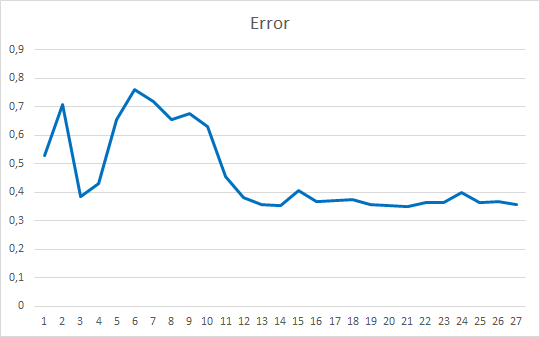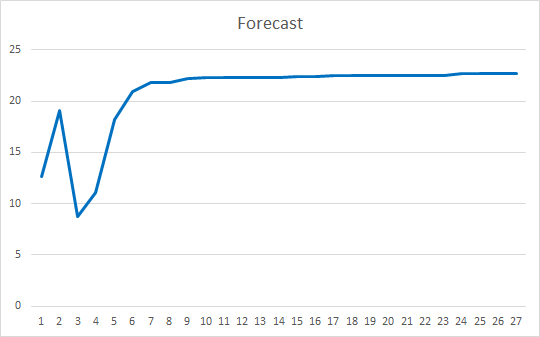### 本文中用到的程序

# 名称 类型 说明
1 Fractal_OCL_Attention.mq5  智能交易系统 含有采用自关注机制的分类神经网络（输出层中有 3 个神经元）的智能交易系统
2 NeuroNet.mqh 类库 用于创建神经网络的类库
3 NeuroNet.cl 代码库 OpenCL 程序代码库

MQL5.zip (489.86 KB)

#### 该作者的其他文章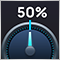开发自适应算法（第一部分）：寻找基本模式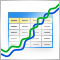使用电子表格建立交易策略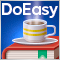DoEasy 函数库中的时间序列（第五十八部分）：指标缓冲区数据的时间序列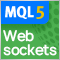MetaTrader 5 中的 WebSockets